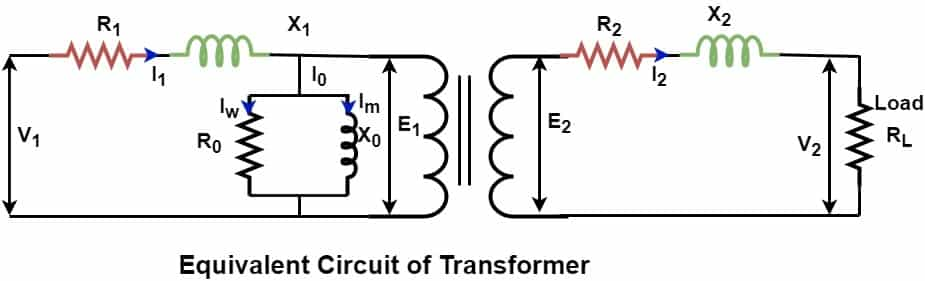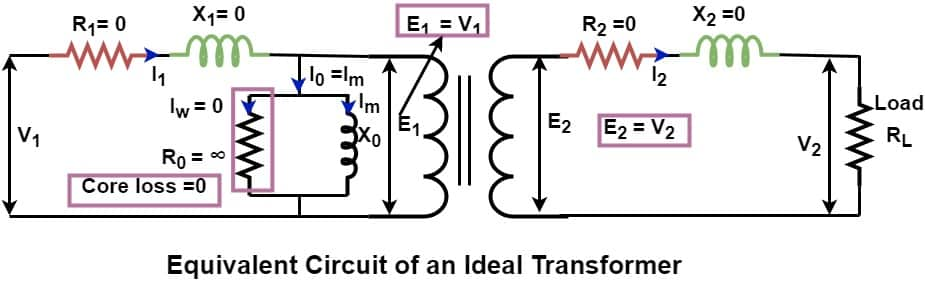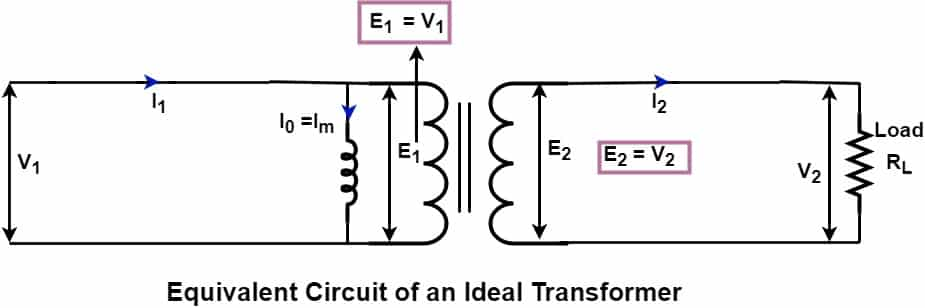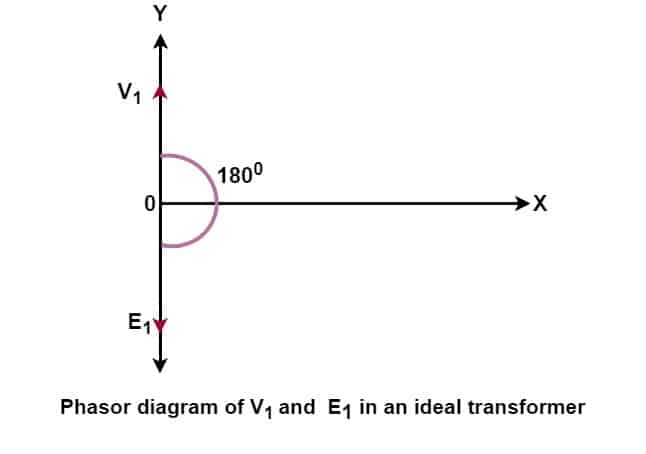# Ideal Transformer-Properties, Phasor Diagram, Working

## What is an Ideal Transformer?

A transformer which is free from all the losses is called an ideal transformer. It has  zero copper loss, zero iron loss and therefore 100% efficient. In an ideal transformer, the output power is equal to the input power in an ideal transformer. An ideal transformer can not exist in real condition.

The transformer has two winding – primary and secondary winding. The winding has resistance and the reactance. Therefore, the losses occurs in a practical transformer. However, an ideal transformer has zero losses and 100 % efficiency, which is completely hypothetical condition.
The equivalent circuit of a practical transformer is as given below.The resistance and reactance of the winding must be as minimum as possible to have low power losses. However, it is only imaginary that winding has zero resistance and reactance.

## Properties of an Ideal Transformer

The proprieties of an ideal transformer are follows.

• Zero Copper loss – Copper loss of an ideal transformer is zero because the winding resistance of the primary and the secondary is zero. This means that both the winding have zero resistance and  thus, copper loss I2R is zero
• Zero leakage flux – The leakage flux is  part of the main flux which does not link to secondary winding of the transformer. In an ideal transformer, we assume that the  entire flux produced in the primary links to the secondary, and thus the entire flux produced in the primary links to the primary and secondary winding. In an ideal transformer main flux is restricted in the core only and there is no flux leakage.
• Zero Iron loss – It is assumed that an ideal transformer has a core of infinite permeability and have infinite resistance. Therefore, no load loss is zero.
• Low Magnetizing Current- An ideal transformer draws very low current to set up magnetic flux in the core. An ideal transformer has infinite permeability core.
• Zero % Voltage Regulation- There is no variation in the secondary output voltage with  current from zero to full load secondary current. Therefore, an ideal transformer has  zero % voltage regulation.
• 100% Efficiency –  If a transformer fulfills above three criteria the losses- eddy current, hysteresis and copper loss is zero and the transformer delivers output power equal to the input power, and efficiency of the transformer is 100 %. However, this is hypothetical condition and transformer  or any equipment can not be 100 % efficient.

Therefore, we can define an ideal transformer as a transformer which has no copper loss, no iron loss and therefore, 100 % efficient.

## Equivalent Circuit of  Ideal Transformer

The equivalent circuit of an ideal transformer is as given below.An ideal transformer has following value of  quantities.

• Primary resistance – R1=0
• Primary reactance – X1 =0
• Magnetizing reactance – X0 Pure inductive
• Core loss component -R0 =∞ [ Core loss- Iw2R= 0 , because Iw =0 ]
• Secondary resistance – R2=0
• Secondary reactance – X2 =0

## Working principle of Ideal Transformer

We can redraw the equivalent circuit diagram of an ideal transformer taking all the transformer quantities  as mentioned above for an ideal transformer.When we feed AC supply V1 to primary of a transformer, the primary winding induces back EMF E1. In an ideal transformer the magnitude of E1 is equal to the applied voltage V1 because transformer has zero winding resistance and leakage reactance.  The alternating voltage cause alternating current to flow in the primary. The rate of change of current cause magnetic flux production. The magnetic flux flow in the core and it links to the secondary. The voltage induced in the primary and secondary winding depends on;
• Number of turns in the primary and secondary
• Frequency of the supply voltage
• Rate of change of flux
Voltage induced in the primary winding is;
E1  =4.44 N1 f Фm  ————–(1)
Voltage induced in the primary winding is;
E2  =4.44 N2 f Фm  ————-(2)
E2 /E1 = N2 /N1 —————–(3)

Where,
E1     = Induced EMF in primary winding
E2     = Induced EMF in secondary winding
N1     = Number of turns in primary winding
N2     =  Number of turns in the secondary winding
f        =  Frequency
Фm   =  Flux in the core

### Ideal Transformer Equations

The losses in an ideal transformer is zero. Therefore input power is equal to the output power.

Input Power = Output Power

P1 = P2
E1 I1 Cos Ф = E2 I2 Cos Ф
E1 I1  = E2 I2
E2 /E1 = I1 / I———–(4)

From equation(3) & (4)

E2 /E1 = I1 / I = N2 /N1  ——-(5)

## Phasor Diagram of Ideal Transformer

When we apply voltage V1 to primary, the back EMF (E1) induced across the primary which opposes the primary voltage. The magnitude of E1 is equal to V1 and polarity of E1 is just 180 degree opposite to V1. The back EMF E1 oppose the applied voltage. The phasor diagram of  V1 and E1 is  given below.The transformer draws more current at the instant of switching on of the transformer because the back EMF induced in the primary is zero.

The transformer draw magnetizing current to set up magnetic field in the transformer core. The magnetizing current lags the applied voltage by 90 degree. This current is called the magnetizing current Iμ . The phasor diagram of  V1 and Iμ is  given below.The alternating magnetizing current set up the alternating flux in the core, and the flux produced is proportional to the magnitude of the magnetizing current and it is  in the phase with the current. The flux produced in the primary gets linked to the secondary and induce E2 voltage. The induced voltage  E2 in the secondary is equal to the output voltage  V2 of the secondary. The phasor diagram of V1, E1, E2 and Iμ is given below.Related Posts
1. Why is the core of a power transformer grounded?
2. Dry Type Transformers: Types and Applications
3. Why PS Class Current Transformer is used for Differential Protection?
4. What is the disadvantage of large exciting current in a transformer?
5. What is the Insulation Class of Transformer oil?